## 4.1.6 The Lattice Heat Flow Equation

Self-heating effects are accounted for by including the lattice heat flow equation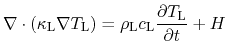(4.22)

in the system of partial differential equations.and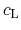are the mass density and the specific heat, respectively. The heat generation term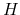depends on the transport model used.

Due to the assumption that the carrier temperature does not deviate from the lattice temperature in the DD model, the energy relaxation times are zero and the heat generation reads: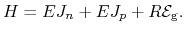(4.23)

The last term in (4.23) gives the recombination heat transferred to the lattice and is proportional to the net recombinationand the band gap eneregy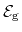.

In the HD transport model, the heat generation is obtained by substituting the local energy balance equations (here only for electrons):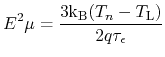(4.24)

in the DD heat generation term (4.23). The resulting expression reads:(4.25)

This solution is obtained from the stationary energy flux balance and the carrier continuity equations (4.13), (4.14). The first term approximates the energy relaxation of the scattering terms of the Boltzmann equation .

An alternative global self-heating model also exists . By calculating a spatially constant lattice temperature it delivers similar results to the standard model, at greatly reduced computational expenses.

S. Vitanov: Simulation of High Electron Mobility Transistors